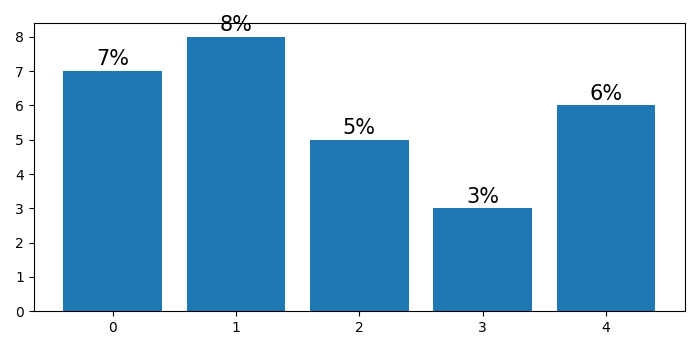# How to change Bar Chart values to percentages in Matplotlib?

To change bar chart values to percentage in matplotlib, we can take the following steps

## Steps

• Set the figure size and adjust the padding between and around the subplots.

• Make a list of frequencies.

• Create a new figure or activate an existing figure.

• Make a bar plot using bar() method.

• Iterate the bar plot and find the height of each patch and use annotate() method to put values in percentages.

• To display the figure, use Show() method.

## Example

import numpy as np
from matplotlib import pyplot as plt

plt.rcParams["figure.figsize"] = [7.00, 3.50]
plt.rcParams["figure.autolayout"] = True

frequencies = [7, 8, 5, 3, 6]

plt.figure()

p1 = plt.bar(np.arange(len(frequencies)), frequencies)

for rect1 in p1:
height = rect1.get_height()
plt.annotate( "{}%".format(height),(rect1.get_x() + rect1.get_width()/2, height+.05),ha="center",va="bottom",fontsize=15)

plt.show()


## Output

It will produce the following output −